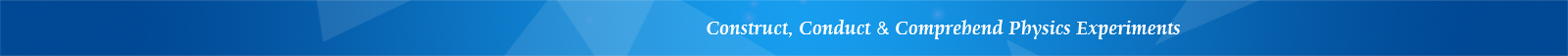## LEMI-40 Apparatus of Magnetic Damping and Kinetic Friction Coefficient

Features

• Reliable and easy-to-use

• Consistent and accurate

• Affordable

Introduction

Magnetic damping is an important concept in electromagnetism. The mechanical effect of magnetic damping has very important applications. This experimental apparatus is designed to measure the uniform sliding speed of a magnetic slide on a non-ferromagnetic conductor inclined rail. Through data processing, magnetic damping coefficient and sliding friction coefficient are acquired. This experimental apparatus is related to physics concepts such as mechanics and electromagnetism.

This apparatus has the following advantages:

1. It has reliable design and convenient angle adjustment.

2. Experimental data has good repeatability and consistency, and the experimental error is small.

3. The incline angle can be easily calculated by directly reading the length of one side of the triangle from the scale on the horizontal support (the other two sides are known).

4. The timer is intelligent and can store 10 counts of timing data.

5. It can be used for general physics experiments in colleges.

Using this apparatus, the following experiments can be conducted:

1. Observe magnetic damping phenomenon, and understand the concept and applications of magnetic damping

2. Observe sliding friction phenomena, and understand the application of friction coefficient in industry

3. Learn how to process data to transfer a nonlinear equation into a linear equation

4. Acquire magnetic damping coefficient and kinetic friction coefficient